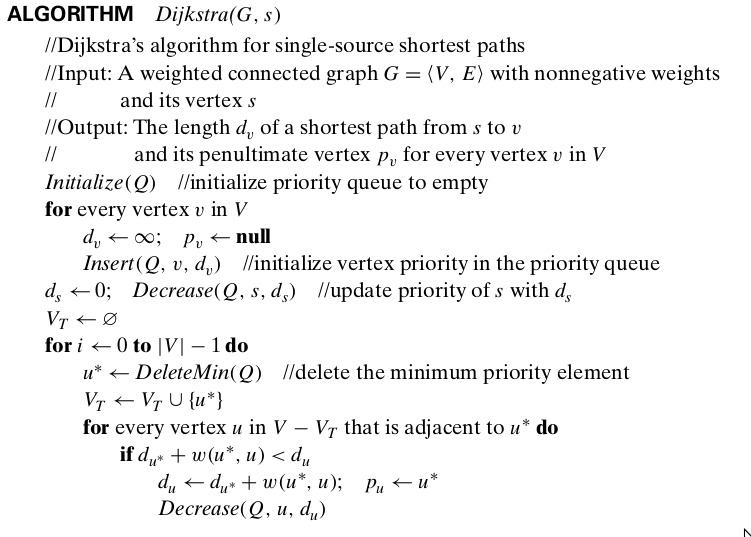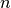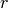# Tree Growth based Graph Algorithms¶

These class of algorithms takes a Graph as input, and generates Tree, which consists of some of edges of input Graph, which are selected according to particular criteria. Some examples are

• DFS
• BFS
• Minimum Spanning Tree Problem (Prim’s and Kruskal’s Algorithm)
• Single Source Shortest Path Problem (Dijkstra’s Algorithm)

## Standard import statement¶

In :

import openanalysis.tree_growth as TreeGrowth


## Implementation Notes¶

• The algorithm should be implemented as a method
• The algorithm works on a networkx graph
• All algorithms start building the tree from a given source, But if source is not given, select source as the first node of Graph
def algorithm_name(G,source = None):
if source is None:
source = G.nodes()
# do other work now

• As soon as node v is visited from node u, yield the tuple containing them
# Assume that visiting is done
yield (u,v)

• To make your life easy, some data structures which comes handy while working with Graphs are included in OpenAnalysis.base_data_structures

## Example - Dijkstra’s Algorithm¶

Dijkstra’s Algorithm finds minimum spanning tree of a graph in greedy manner. The algorithm is given belowAlgo

## Implementation¶

Since we need a Priority Queue here, Let’s import it

In :

from openanalysis.base_data_structures import PriorityQueue


Now, Let’s implement the algorithm

In :

def dijkstra(G, source=None):                 # This signature is must
if source is None: source = G.nodes()  # selecting root as source
V = G.nodes()
dist, prev = {}, {}
Q = PriorityQueue()
for v in V:
dist[v] = float("inf")
prev[v] = None
dist[source] = 0
visited = set()
for i in range(0, len(G.nodes())):
u_star = Q.remove_min()
if prev[u_star] is not None:
yield (u_star, prev[u_star])    # yield the edge as soon as we visit the nodes
for u in G.neighbors(u_star):
if u not in visited and dist[u_star] + G.edge[u][u_star]['weight'] < dist[u]:
dist[u] = dist[u_star] + G.edge[u][u_star]['weight']
prev[u] = u_star


Note how implementation looks similiar to the algorithm, except the if block, which is used to yield the edges.

## Visualizing the Algorithm¶

• apply_to_graph(fun): Creates Random Geometric Graph of 100 nodes and applies fun on it to build the tree. After building the tree, it shows original graph and the tree side by side
• tree_growth_visualizer(fun): Creates Random Geometric Graph of 100 nodes and applies fun on it to build the tree. Saves the animation of building the tree in output/ folder

## Random Geometric Graph¶

Random Geometric Graph is created using two parameters. Number of nodes, and radiuus.points are chosen randomly on plane. The edge between 2 nodes is created if and only if the distance between 2 nodes is less thanimport networkx
G = nx.random_geometric_graph(100,2.3) # n,r
pos = nx.get_node_attribute('pos')

In :

TreeGrowth.apply_to_graph(dijkstra)After executing

TreeGrowth.tree_growth_visualizer(dijkstra)


go to output/ directory to see the mp4 files

## Example File¶

You can see more examples at Github